# NCERT Solutions for Class 9 Science Sound part 1## myCBSEguide App

CBSE, NCERT, JEE Main, NEET-UG, NDA, Exam Papers, Question Bank, NCERT Solutions, Exemplars, Revision Notes, Free Videos, MCQ Tests & more.

NCERT solutions for Science Sound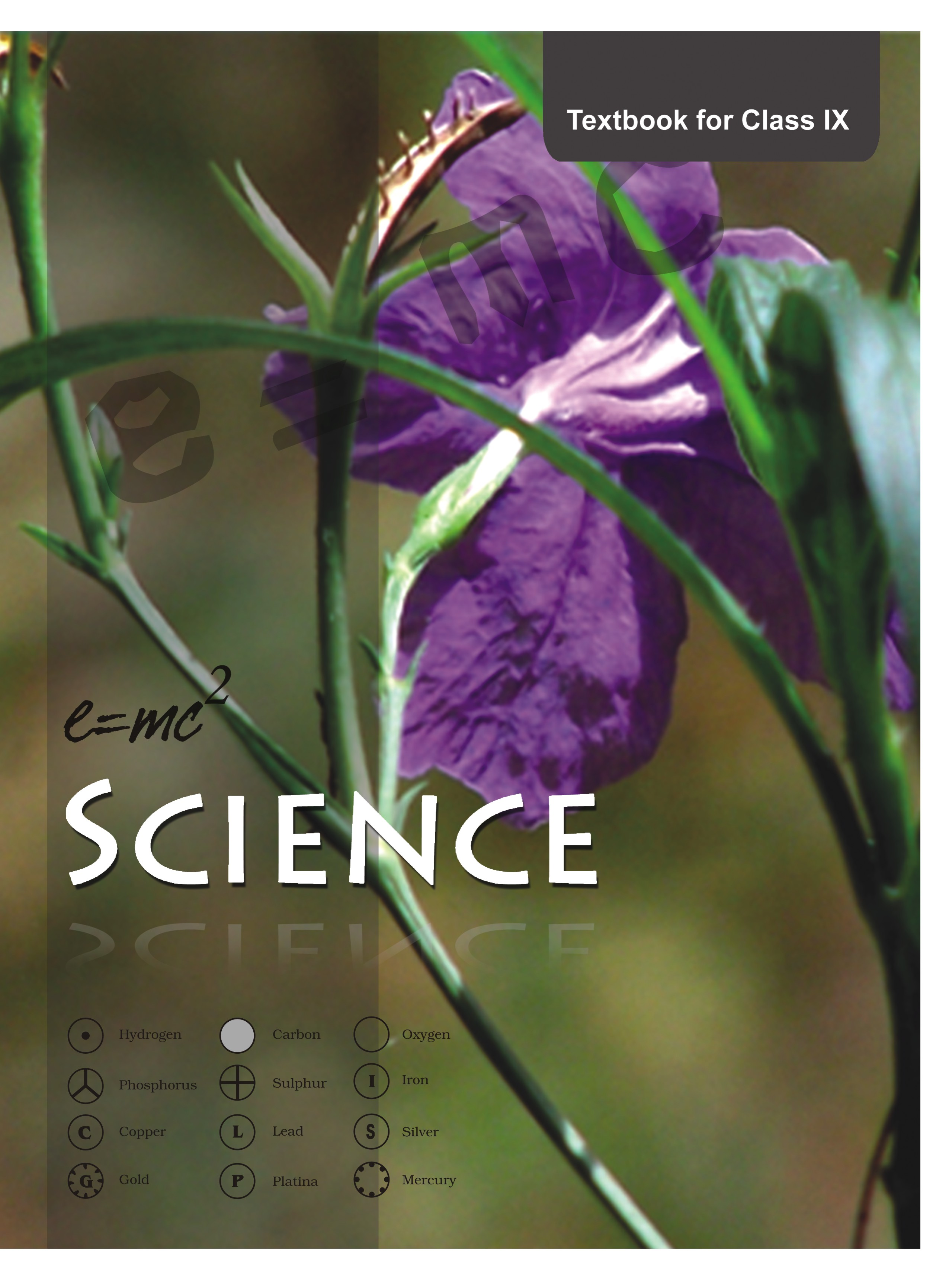## NCERT Class 9 Science Chapter wise Solutions

• 01 –  Matter in Our Surroundings
• 02 – Is Mattter Around us Pure
• 03 – Atoms and Molecules
• 04 – Structure of the Atom
• 05 – The Fundamental Unit of Life
• 06 – Tissues
• 07 – Diversity in Living Organisms
• 08 – Motion
• 09 – Force and Laws of Motion
• 10 – Gravitation
• 11 – Word and Energy
• 12 – Sound
• 13 – Why Do We Fall Ill
• 14 – Natural Resources
• 15 – Improvement in Food Resources

## NCERT Solutions for Class 9 Science Sound part 1

###### 1. How does the sound produced by a vibrating object in a medium reach your ear.

Ans. As we speak, the particles of air near our mouth are pushed forward so they get compressed. Then they compress the other particles of air. As the compression proceeds the particles of air near our mouth expand again and thus rarefaction occurs. This process is repeated further and as a result sound wave propagates in the form of compressions and rarefactions to the listener’s ear.

NCERT Solutions for Class 9 Science Sound part 1

###### 1. Explain how sound is produced by your school bell.

Ans. When the peon strikes the school bell with a hammer, the particles of bell metal start vibrating and those vibrations produce sound.

###### 2. Why are sound waves called mechanical waves?

Ans. Since sound waves need a medium for their propagation therefore we can say that sound waves are mechanical waves.

###### 3. Suppose you and your friend are on the moon. Will you be able to hear any sound produced by your friend?

Ans. There is no air on moon hence there is no medium for sound propagation on moon. As a result, me and my friend will not be able to hear any sound produced by my friend.

NCERT Solutions for Class 9 Science Sound part 1

###### 1. Which wave property determines

(a) loudness,

(b) pitch?

Ans. (a) The amplitude of the wave determines loudness of sound.

(b) The frequency of the wave determines pitch of sound.

###### 2. Guess which sound has a higher pitch: guitar or car horn?

Ans. Sound of Guitar has a higher pitch.

###### 1. What are wavelength, frequency, time period and amplitude of a sound wave?

Ans. wavelength: For a sound wave, the combined length of a compression and an adjacent rarefaction is called its wavelength even the distance between centres of two consecutive compressions or two consecutive rarefactions is also equal to its wavelength.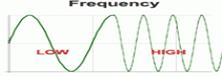frequency: The number of vibrations or oscillations per second is called frequency i.e. it is the number of complete waves or cycles produced in one second.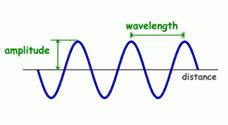time period: The time taken to complete one vibration/oscillation/complete wave is called time period. It is measured in seconds.

amplitude: It is the maximum displacement of the particles of the medium from their mean/original position at rest.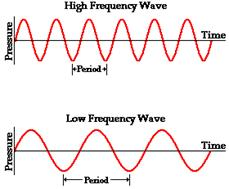###### 2. How are the wavelength and frequency of a sound wave related to its speed?

Ans. From the equation: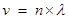where v = velocity/speed

n = frequency of wave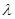= wavelength of wave

NCERT Solutions for Class 9 Science Sound part 1

###### 3. Calculate the wavelength of a sound wave whose frequency is 220 Hz and speed is 440 m/s in a given medium.

Ans. Since we know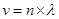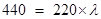= 440/220 = 2 m

###### 4. A person is listening to a tone of 500 Hz sitting at a distance of 450 m from the source of the sound. What is the time interval between successive compressions from the source?

Ans. The time interval between successive compressions from the source

T = 1/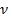= 1/500 = 0.002 second.

###### 1. Distinguish between loudness and intensity of sound.

Ans.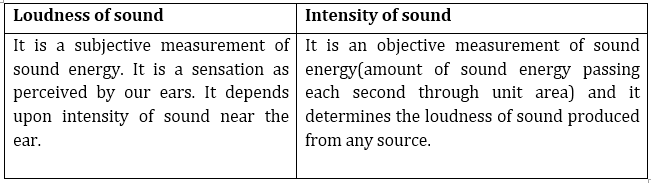NCERT Solutions for Class 9 Science Sound part 1

###### 1. In which of the three media, air, water or iron, does sound travel the fastest at a particular temperature?

Ans. Sound will travel the fastest in iron at a particular temperature.

###### 1. An echo returned in 3 s. What is the distance of the reflecting surface from the source, given that the speed of sound is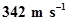?

Ans. Speed of sound = distance/time

therefore, distance travelled by sound during echo =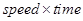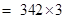= 1026 m

so the distance of reflecting surface = 1026/2 =513 m

NCERT Solutions for Class 9 Science Sound part 1

###### 1. Why are the ceilings of concert halls curved?

Ans. The ceilings of concert halls are curved because such architecture helps the sound to reach all the corners and places of concert hall.

NCERT Solutions for Class 9 Science Sound part 1

###### 1. What is the audible range of the average human ear?

Ans. 20 Hz to 20,000Hz.

###### 2. What is the range of frequencies associated with

(a) Infrasound?

(b) Ultrasound?

Ans. Infrasound = less than 20 Hz

Ultrasound = greater than 20 KHz

NCERT Solutions for Class 9 Science Sound part 1

###### 1. A submarine emits a sonar pulse, which returns from an underwater cliff in 1.02 s. If the speed of sound in salt water is 1531 m/s, how far away is the cliff?

Ans. Distance travelled by a sonar pulse = speed of sound in salt water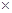time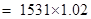= 1561.62 m

therefore, the distance of cliff from submarine = 1561.62/2 = 780.81 m

## NCERT Solutions for Class 9 Science

NCERT Solutions Class 9 Science PDF (Download) Free from myCBSEguide app and myCBSEguide website. Ncert solution class 9 Science includes text book solutions .NCERT Solutions for CBSE Class 9 Science have total 15 chapters. 9 Science NCERT Solutions in PDF for free Download on our website. Ncert Science class 9 solutions PDF and Science ncert class 9 PDF solutions with latest modifications and as per the latest CBSE syllabus are only available in myCBSEguide.

## CBSE app for Class 9

To download NCERT Solutions for class 9 Science, Computer Science, Home Science,Hindi ,English, Maths Social Science do check myCBSEguide app or website. myCBSEguide provides sample papers with solution, test papers for chapter-wise practice, NCERT solutions, NCERT Exemplar solutions, quick revision notes for ready reference, CBSE guess papers and CBSE important question papers. Sample Paper all are made available through the best app for CBSE students and myCBSEguide website.1. Thanks